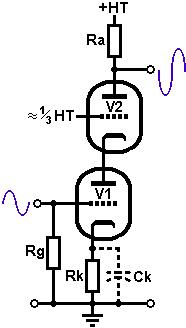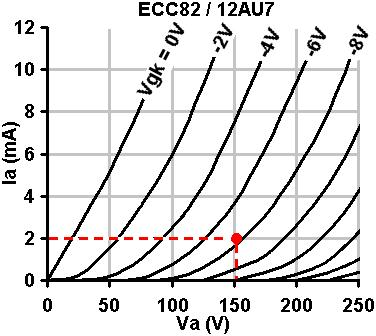# The Valve Wizard

How to design valve guitar amplifiers!

The Cascode

The cascode is a means of using a pair of triodes to achieve very high levels of gain and input sensitivity from a single stage. For guitar amps it can be imagined to be a simulation of a pentode, and indeed the circuit does resemble one. It has high gain, high ra and very low input capacitance just like a pentode, but without the inherent drawbacks of noise, microphonics and expense. The two triodes are usually of the same type for the sake of simplicity and convenience, but they do not have to be. Hi gm triodes provide the most gain, and the ECC82 or 6SN7 work remarkably well as cascodes. The ECC83 will work to, though it is a little more fiddly to set up.The operation of the circuit is simple: The lower triode amplifies in the normal way. The amplified (and inverted) signal is passed from the lower anode to the cathode of the upper valve. The signal voltage on the cathode then modulates the current flowing through upper triode, allowing the signal to be amplified yet again (non-inverting this time). The grid of the upper valve is kept at a fixed voltage (more or less) to provide the correct bias for the upper triode. Overall the output signal in out of phase with the input.
The anode load of the lower triode consists of the cathode of the upper triode. The upper cathode has a low AC impedance, so the gain of the lower triode is also low (rather like using a very low anode resistor in a normal gain stage) so it acts mainly as a voltage-to-current converter. The upper triode has a normal resistive anode load, and so has fairly normal level of gain and does most of the work. In this way, the gain of the lower triode is multiplied by the gain of the upper stage, resulting in a very high overall level of gain!

There is more than one way to go about designing a cascode, but this is how I do it. Firstly, a cascode's characteristics look a lot like a pentode, except that they are shifted to the right by an amount equal to the lower triode's quiescent anode voltage, as seen in the oscillograph. The lower triode's anode voltage is more-or-less equal to the upper triodes grid voltage, which we will call the 'screen grid', Vg2,for simplicity.The higher the screen voltage, the higher the gm and therefore the gain, but the lower the output signal swing, because the curves move further towards the right. The peak-to-peak output signal swing is limited to HT-Vg2. With most valves, a screen voltage in the region of 80V seems to be ideal, although with low gm valves it may need to be higher.
As with the pentode, the load line should pass through or slightly below the 'knee' for the most 'pentode-like' tone. If it passes above the knee we will get a more rounded, triode tone (albeit a high-gain triode).

We begin by looking at the normal anode-characteristics of the LOWER triode. We will assume that the screen voltage is equal to the anode voltage of the lower triode. Draw a line from this voltage up to the 0V grid curve; this indicates where the 'knee' will occur. Knowing the HT we can now choose a load and draw a load line in the usual way, to see if it passes through, above or below the knee, and adjust the screen voltage if necessary. In this case I have chosen an ECC82, and HT of 300V and a tentative screen voltage of 55V. It is clear that a 47k load will pass right through the knee (I adjusted the screen voltage so it would, of course! You may prefer to make the load line pass below the knee, for an even more 'pentode-ish' sound). Note, the blue line is NOT the actual load line that the lower triode will operate on, it's merely a design aid.

The bias point of the lower triode must lie on the vertical red line. For centre bias, it will be half-way up of course. Wherever you decide to put it, read off the gm (transconductance). In this case it is about 1.4mA/V. Because the lower triode generates all the current swing, its gm decides the gm of the whole cascode, and this allows us to immediately predict the gain, which is the same as for a pentode:
A = gmRa
A = 0.0014 x 47000
= 66. That's about the same as a single ECC83 triode, but we're getting it from an ECC82, and it should sound a bit like a pentode! In practice the gain may be a little lower, but not much.Biasing the Lower Triode: This is done in the usual way. Looking at the chosen bias point we see that the bias voltage is about -2V and the anode current is2mA. Applying Ohm's law:
Rk = V/I = 2 / 0.002 = 1k
The cathode bypass capacitor has hardly any effect on the output impedance of the cascode, but it does affect the gain, a lot! For most purposes it is sufficient to choose the cathode bypass capacitor according to:
Ck = 1/ (2 . pi . f . Rk)
Where f is the lowest frequency at which we want full gain. In this case, a generic value of 22uF will cover the whole audio range, but you may prefer to use a smaller value to cut the bass.All that remains is to bias the upper triode. From the chosen bias point above, we can see that the quiescent current is 2mA. The voltage dropped across the anode resistor must therefore be 0.002 * 47000 = 94V. The voltage across the lower triode is 55V, so that leaves:
300 - 94 - 55 = 151V
across the upper triode. Looking the the normal anode characteristics of the upper triode we can plot this information to find its bias point. In this case it indicates a bias of about -8V. The screen-grid voltage must therefore be 8V below the anode of the lower triode, at 55 - 8 = 47V.

Fixed Biasing the Upper Triode: The textbook arrangement is to feed the screen grid from a potential divider from the HT. The upper grid does not draw any current, so we require only a voltage reference and as such, R1 and R2 can be made high in value so as to present a negligible load to the power supply. If we choose a value of 560k for R1 and apply the formula for a voltage divider we can find R2:R2 = R1 / (HT - Vg2) * Vg2
R2 = 560k / (300 - 47) * 47
= 104k
The nearest standard of 100k is close enough. The actual grid-leak value is equal to R1 and R2 in parallel, which is 85k (check the data sheet for the maximum allowable for your chosen valve). This value is used to find a suitable decoupling capacitor. So, for a low roll-off of 20Hz:
Cg2 = 1 / (2 * pi * f * Rg)
Cg2 = 1 / (2* pi * 20 * 85000)
= 93nF
100nF is the nearest standard, and the completed circuit is shown on the right with measured voltages. They are not too far away from the design values!

Alternatively, a string of zener diodes could set the grid voltage rock-solid. A bypass capacitor should still be used to shunt zener noise though.

Grid-leak biasing the Upper Triode: Alternatively we can create a self-biased version by simply using a grid-leak resistor on the upper triode, and a large grid-bypass capacitor. At quiescence, the upper triode is zero-biased of course, but when we input a signal a charging current flows into the capacitor via the grid-leak, which lowers the voltage on the screen grid. The larger the signal, the greater the bias developed, which creates a subtle compression effect on sustained passages, rather like power-supply sag. This method is simple and works well for guitar.

The grid leak should be a typical value, say 1Meg. The time constant produced by Rg2 and Cg2 should be at least equal to the time period of the lowest frequency which is to be amplified at full gain. The value of Cg2 may therefore be chosen according to:Cg2 = (1/f)/Rg2
For a low-frequency of 10Hz,
Cg2 = (1/10)/1000000 = 100nF

Note that the measured quiecent voltages will different from the expected -as shown by the completed circuit on the right- because the upper triode is zero biased. Only under full drive will the average current fall and the voltages shift back towards the expected values.

Output impedance: Assuming the cathode is bypassed, the cascode has a total ra approximately equal to:
ra(cascode) = ra1 * (mu2 + 1)
So the output impedance will be:
Zout = Ra2 || ra(cascode)
But since ra(cascode) is usually large compared to Ra1, this simplifies to:
Zout = Ra1, or 47k in this case.

Total Gain: The gain of the cascode is equal to:
A = 1 / (1/gm1 * Ra2) + ((ra2 + Ra2)/Ra2) * (1/ mu1 (mu2 + 1))
When using two identical triodes this can be simplified to:
A = gm1 * Ra2
as was calculated earlier.

Heater considerations: Since the cathode of the upper triode is at a high voltage, it may be necessary to elevate the heater supply to avoid exceeding the maximum rated heater to cathode voltage. In this case it is not necessary, but it is always worth checking.